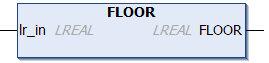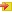# FLOORThe FLOOR function determines an integral value from a floating point number that is a fraction smaller than or equal that number. The resulting number is of type `LREAL` and is therefore not limited to the value range of integer variables.

Examples

FLOOR(2.8) = 2

FLOOR(-2.8) = -3

Similar functions: TRUNC, LTRUNCUnlike FLOOR, the LTRUNC function always determines the integral part of a number. For positive values, this number is smaller than or equal the input parameter, for negative values it is greater than or equal the input parameter.

##Inputs

`VAR_INPUT    lr_in : LREAL;END_VAR`

Name

Type

Description

lr_in

LREAL

Function parameters of type LREAL

## Requirements

Development environment

Target system type

PLC libraries to include

TwinCAT v3.0.0

PC or CX (x86)

Tc2_Math